Lakhmir Singh & Manjit Kaur: Metals and Non-metals, Solutions- 3

# Lakhmir Singh & Manjit Kaur: Metals and Non-metals, Solutions- 3 - Science Class 10

(Page No - 167)

Question 1:
What is the name of the chemical bond formed :
(a) by the sharing of electrons between two atoms ?
(b) by the transfer of electrons from one atom to another ?
Solution :

(a) Covalent bond.
(b) Ionic bond .

Question 2:
Name a carbon containing molecule which has two double bonds.
Solution :

Carbon dioxide

Question 3:
What would be the electron-dot structure of carbon dioxide which has the formula C02 ?
Solution :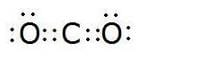Question 4:
What type of chemical bond is formed between :
(a) potassium and bromine ?
(b) carbon and bromine ?
Solution :

(a) Ionic bond.
(b) Covalent bo nd.

Question 5:
(a) What do we call those particles which have more or less electrons than the normal atoms ?
(b) What do we call those particles which have more electrons than the normal atoms ?
(c) What do we call those particles which have less electrons than the normal atoms ?
Solution :

(a) Ions
(b) Anions
(c) Cations

Question 6:
(a) The atomic number of sodium is 11. What is the number of electrons in Na+ ?
(b) The atomic number of chlorine is 17. What is the number of electrons in Cl ?
Solution :

(a) No. of electrons in Na+ = 10
(b) No. of electrons in Cl = 18

Question 7:
The atomic number of an element X is 8 and that of element Y is 12. Write down the symbols of the ions you would expect to be formed from their atoms.
Solution :

X will form X2-
Y will form Y2+

Question 8:
(a) Write down the electronic configuration of (i) magnesium atom, and (ii)magnesium ion. (At. No. of Mg = 12)
(b) Write down the electronic configuration of (i) sulphur atom, and (ii) sulphide ion. (At. No. of S = 16)
Solution :

(a) (i) E.C of Mg = 2, 8, 2 (ii) E.C of Mg2+ = 2, 8
(b) (i) E.C of S = 2, 8, 6 (ii) E.C of S2- = 2, 8, 8

Question 9:
What type of chemical bonds are present in a solid compound which has a high melting point, does not conduct electricity in the solid state but becomes a good conductor in the molten state ?
Solution :

Ionic bonds .

Question 10:
State whether the following statement is true or false :
The aqueous solution of an ionic compound conducts electricity because there are plenty of free electrons in the solution.
Solution :

False (It should be ‘ions’ in place of ‘electrons’) .

Question 11:
What type of bonds are present in hydrogen chloride and oxygen ?
Solution :

Covalent bond

Question 12:
Write the electron-dot structures for the following molecules :
(i) NaCl (ii) Cl2
Solution :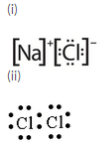Question 13:
What type of bonds are present in water molecule ? Draw the electron-dot structure of water (H20).
Solution :

Covalent bonds are present in a water molecule. Electron dot structure of water: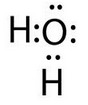Question 14:
What type of bonds are present in methane (CH4) and sodium chloride (NaCl) ?
Solution :

Methane: Covalent bonds
Sodium chloride: Ionic bonds

Question 15:
State one major difference between covalent and ionic bonds and give one example each of covalent and ionic compounds.
Solution :

Ionic compounds conduct electricity when dissolved in water or melted whereas covalent compounds do not conduct electricity.
Ionic compound – NaCl
Covalent compound – Carbon Dioxide

Question 16:
What type of bonds are present in the following molecules ? Draw their electron-dot structures.
(i) H2 (ii) CH4 (iii) Cl2 (iv) O2
Solution :

Covalent bonds are present in the given molecules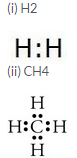.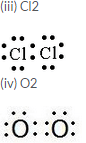Question 17:
Which inert gas electron configuration do the Cl atoms in Cl2 molecule resemble ? What is this electron configuration ?
Solution :

Argon; E.C = 2, 8,8

Question 18:
Which of the following compounds are ionic and which are covalent ?
Urea, Cane sugar, Hydrogen chloride, Sodium chloride, Ammonium chloride, Carbon tetrachloride, Ammonia, Alcohol, Magnesium chloride.
Solution :

Ionic compounds: Sodium chloride, Ammoniu m chloride, Magnesium chloride.
Covalent compounds:
Urea, Cane sugar, Hy drogen chloride,
Carbon tetrachloride, Ammonia, Alcohol .

Question 19:
Give one example each of the following :
(i) Amolecule containing a single covalent bond
(ii) Amolecule containing a double covalent bond
(iii) Amolecule containing a triple covalent bond
(iv) Acompound containing an ionic bond
Solution :

(i) Hydrog en
(ii) Oxygen
(iii) Nitrogen
(iv) Sodium chloride

(Page No - 168)

Question 20:
Fill in the blanks in the following sentences :
(i) Two atoms of the same element combine to form a molecule. The bond between them is known as…………………………………bond.
(ii) Two chlorine atoms combine to form a molecule. The bond between them is known as
(iii) In forming oxygen molecule,………….. electrons are shared by each atom of oxygen.
(iv) In forming N2 molecule,……….. electrons are shared by each atom of nitrogen.
(v) The number of single covalent bonds in C2H2 molecule are…………………………
(vi) Melting points and boiling points of ionic compounds are generally………………… than those of covalent compounds.
Solution :

(i) Covalent
(ii) Covalent
(iii) Two
(iv) Three
(v) Two
(vi) Higher

Question 21:
(a) What is a covalent bond ? What type of bond exists in (i) CCl4, and (ii) CaCl2 ?
(b) What is an ionic bond ? What type of bond is present in oxygen molecule ?
Solution :

(a) The chemical bond formed by the sharing of electrons between two atoms is known as a covalent bond.
(i) Covalent bonds (ii) Ionic bonds
(b) The chemical bond formed by the transfer of electrons from one atom to another is known as an ionic bond.
Covalent bond is present in an oxygen molecule.

Question 22:
(a) What is an ion ? Explain with examples.
(b) What is the nature of charge on (i) a cation, and (ii) an anion ?
(c) Name the cation and anion present in MgCl2. Also write their symbols.
Solution :

(a) An ion is an electrically charged atom (or group of atoms). Example: Sodium ion, Na + , magnesium ion, Mg 2+ .
(b) (i) Positive charge (ii) Negative charge
(c) Cation: Magnesium ion, Mg 2+
Anion: Chloride ions, 2Cl

Question 23:
(a) What type of chemical bond is present in chlorine molecule ? Explain your answer.
(b) Explain the formation of a chlorine molecule on the basis of electronic theory of valency.
Solution :

(a) Covalent bond ; Because whenever two atoms of the same element combine to form a molecule, a covalent bond is formed.
(b) The atomic number of chlorine is 17, so its electronic configuration is 2,8,7. Chlorine atom has 7 electrons in its outermost shell and needs 1 more electron to complete its octet and become stable.
It gets this electron by sharing with another chlorine atom. So, two chlorine atoms share one electron each to form a chlorine molecule. Because the two chlorine atoms share electrons, there is a strong force of attraction between them which holds them together. This force is called covalent bond.
Now, each chlorine atom in the chlorine molecule has the electronic configuration 2,8,8 resembling its nearest inert gas argon. Since the chlorine atoms in a chlorine molecule have inert gas electron arrangements, therefore, a chlorine molecule is more stable than two separate chlorine atoms.

Question 24:
(a) Giving one example each, state what are (i) ionic compounds, and (ii) covalent compounds.
(b) Compare the properties of ionic compounds and covalent compounds.
Solution :

(a) (i) The compounds containing ionic bonds are known as ionic compounds. They are formed by the transfer of electrons from one atom to another.
Example: Sodium chloride, NaCl.
(ii) The compounds containing covalent bonds are known as covalent compounds. They are formed by the sharing of electrons between atoms. Example: Methane, CH 4 .
(b) Ionic compounds
(i) Ionic compounds are usually crystalline solids.
(ii) Ionic compounds have high melting and boiling points.
(iii) Ionic compounds are usually soluble in water.
Covalent compounds
(i) Covalent compounds are usually liquids or gases.
(ii) Covalent compounds have low melting and boiling points.
(iii) Covalent compounds are usually insoluble in water.

Question 25:
Explain why :
(a) covalent compounds have generally low melting points.
(b) ionic compounds have generally high melting points.
Solution :

(a) Covalent compounds have generally low melting points because they are made up of electrically neutral molecules. So, the force of attraction between the molecules of a covalent compound is ve ry weak. Hence, only a small amount of heat energy is required to break these weak molecular forces.
(b) Ionic compounds are made of up of positive and negative ions. There is a strong force of attraction between the oppositely charged ions, so a lot of heat energy is required to break this force of attraction and melt or boil the ionic compound. Due to this, ionic compounds have high melting points.

Question 26:
(a) Give two general properties of ionic compounds and two those of covalent compounds.
(b) State one test by which sodium chloride can be distinguished from sugar.
Solution :

(a) Ionic compounds:
(i) They have high melting and boiling points.
(ii) They are usually soluble in water.
Covalent compounds:
(i) They have low melting and boiling points.
(ii) They are usually insoluble in water.
(b) An aqueous solution of sodium chloride conducts electricity but a sugar solution does not conduct electri city.

Question 27:
(a) Explain why, ionic compounds conduct electricity in solution whereas covalent compounds do not conduct electricity .
(b) Which of the following will conduct electricity and which not ?
MgCl2, CCl4, NaCl, CS2, Na2S Give reasons for your choice.
Solution
:
(a) Ionic compounds conduct electricity in solution because they are made up of electrically charged ions but covalent compounds are made u p of electrically neutral molecules so they do not conduct electricity.
(b) Conduct electricity: MgCl2 , NaCl, Na2 S (Ionic compounds)
Do not con duct electricity: CCl4 , CS2 ( Covalent compounds) .

Question 28:
(a) Name one ionic compound containing chlorine and one covalent compound containing chlorine.
(b) How will you find out which of the water soluble compound A or B is ionic ?
Solution :

(a) Ionic compound: Sodium chloride, NaCl.
Cova lent compound: Carbon tetrachloride, CCl4
(b) Out of A and B, the compound whose aqueous solution cond ucts electricity will be an ionic compound.

Question 29:
Explain why, a solution of cane sugar does not conduct electricity but a solution of common salt is a good conductor of electricity.
Solution :

Common salt is an ionic compound containing ionic bonds whereas cane sugar is a covalent compound containing covalent bonds. Since, ionic compounds conduct electricity and covalent compounds do not, hence common salt is a good conductor of electricity and cane sugar is a non-conductor of electricity.

Question 30:
Give the formulae of the compounds that would be formed by the combination of the following pairs of elements :
(a) Mg and N2 (b) Li and O2
(c) Al and Cl(d) K and H
Solution :

(a) Mg3 N2
(b) LiO
(c) AlCl3
(d) KH

Question 31:
(a) What are noble gases ? What is the characteristic of the electronic configuration of noble gases ?
(b) What is the cause of chemical bonding (or chemical combination) of atoms of elements ?
Solution :

(a) There are some elements in group 18 of the periodic table which do not combine with other elements. These elements are helium, neon, argon, krypton, xenon and radon . They are known as noble gases or inert gases because they are unreactive.
If we look at the electronic configuration of noble gases, we would notice that except helium, all other inert gases have 8 electrons (helium has 2) in their outermost shells. This is considered to be the most stable arrangement of electrons.
(b) Atoms form chemical bonds to achieve stability by acquiring the inert gas electron configuration.

Question 32:
(i) Write electron-dot structures for magnesium and oxygen.
(ii) Show the formation of MgO by the transfer of electrons.
(iii) What are the ions present in this compound ?
Solution :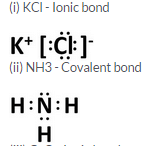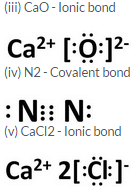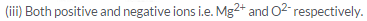Question 33:
Draw the electron-dot structure of a hydrogen chloride molecule :
(i) Which inert gas does the H atom in HCl resemble in electron arrangement ?
(ii) Which inert gas does the Cl atom in HCl resemble in electron arrangement ?
Solution :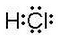(i) Helium(ii) Argon

Question 34:
What type of bonding would you expect between the following pairs of elements ?
(i) Calcium and Oxygen
(ii) Carbon and Chlorine
(iii) Hydrogen and Chlorine
Solution :

(i) Ionic bonding.
(ii) Covalent bonding.
(iii) Covalent bonding.

(Page No - 169)

Question 35:
Describe how sodium and chlorine atoms are changed into ions when they react with each other to form sodium chloride, NaCl. What is the name given to this type of bonding ? (At. No of sodium = 11; At. No. of chlorine = 17)
Solution :

The atomic number of sodium is 11, so its electronic configuration is 2,8,1. Sodium atom has only 1 electron in its outermost shell. So, the sodium atom donates one electron (to a chlorine atom) and forms a sodium ion, Na+ . The atomic number of chlorine is 17, so its electronic configuration is 2,8,7. Chlorine atom has 7 electrons in its outermost shell and needs 1 more electron to achieve the stable 8-electron inert gas configuration. So, a chlorine atom takes one electron (from the sodium atom) and forms a negatively charged chloride ion, Cl- This type of bonding is called ionic bonding.

Question 36:
What is the difference between a cation and an anion ? How are they formed ? Give the names and symbols of one cation and one anion.
Solution :

A positively charged ion is known as cation. A cation is formed by the loss of one or more electrons by an atom. For example: sodium loses 1 electron to form a sodium ion, Na + , which is a cation.
A negatively charged ion is known as anion. An anion is formed by the gain of one or more electrons by an atom. For example: A chlorine atom gains (accepts) 1 electron to form a chloride ion, Cl – , which is an anion.

Question 37:
Using electron-dot diagrams which show only the outermost shell electrons, show how a molecule of nitrogen, N2, is formed from two nitrogen atoms. What name is given to this type of bonding ? (Atomic number of nitrogen is 7)
Solution :

Since nitrogen has 5 electrons in its outermost shell so, to achieve the 8-electron structure of an inert gas, it needs 3 more electrons and hence combines with another nitrogen atom to form a molecule of nitrogen gas.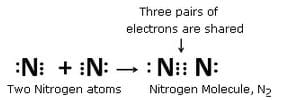This type of bonding is called covalent bonding.

Question 38:
Draw the electron-dot structures of the following compounds and state the type of bonding in each case :
(i) CO2 (ii) MgO (iii) H,O (iv) HCl (v) MgCl2
Solution :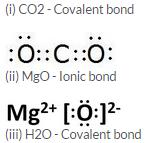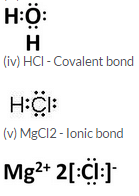Question 39:
Using electron-dot diagrams which show only the outermost shell electrons, show how a molecule of oxygen, O2, is formed from two oxygen atoms. What name is given to this type of bonding ? (At. No. of oxygen = 8)
Solution :

Since an oxygen atom has 6 electrons in its outermost shell so, it needs 2 more electrons to achieve the stable 8-electron inert gas configuration. Hence, it combines with another oxygen atom and forms a molecule of oxygen.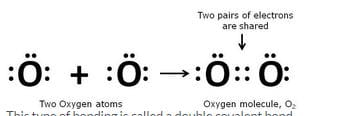This type of bonding is called a double covalent bond.

Question 40:
Draw the electron-dot structures of the following compounds and state the type of bonding in each case :

(i) KCl (ii) NH3 (iii) CaO (iv) N(iv) CaCl2
Solution :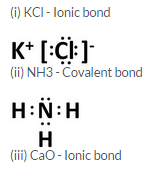Question 41:
Explain why, a salt which does not conduct electricity in the solid state becomes a good conductor in molten state.
Solution :

Although solid ionic compounds are made up of ions but they do not conduct electricity in solid state. This is because in the solid ionic compound, the ions are held together in fixed positions by strong electrostatic forces and cannot move freely. However, when we dissolve the ionic solid in water or melt it, the crystal structure is broken down and ions become free to move and conduct electricity. Thus, an aqueous solution of an ionic compound conducts electricity because there are plenty of free ions in the solution which are able to conduct electric current.

Question 42:
(a) Write down the electronic configuration of (i) sodium atom, and (ii) chlorine atom.
(b) How many electrons are there in the outermost shell of (i) a sodium atom, and (ii) a chlorine atom ?
(c) Show the formation of NaCl from sodium and chlorine atoms by the transfer of electron(s).
(d) Why has sodium chloride a high melting point ?
(e) Name the anode and the cathode used in the electrolytic refining of impure copper metal.
Solution :

(a) (i) Sodium – 2, 8, 1 (ii) Chlorine – 2, 8, 7
(b) (i) Sodium = 1 (ii) Chlorine = 7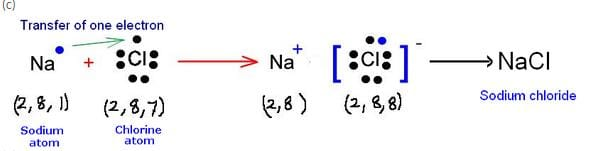(d) Sodium chloride has a high melting point because it is an ionic compound and these compounds are made of up of positive and negative ions. There is a strong force of attraction between the oppositely charged ions, so, a lot of heat energy is required to break this force of attraction and melt or boil the ionic compound.
(e) Anode: Thick block of impure copper metal; Cathode: Thin strip of p ure copper metal

Question 43:
(a) Write the electron arrangement in (i) a magnesium atom, and (ii) an oxygen atom.
(b) How many electrons are there in the valence shell of (i) a magnesium atom, and (ii) an oxygen atom ?
(c) Show on a diagram the transfer of electrons between the atoms in the formation of MgO.
(d) Name the solvent in which ionic compounds are generally soluble.
(e) Why are aqueous solutions of ionic compounds able to conduct electricity ?
Solution :

(a) (i) Magnesium -2, 8, 2
(ii) Oxygen – 2, 6
(b) (i) Magnesium = 2
(ii) Oxygen = 6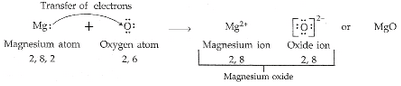(d) Water .

(e) An aqueous solution of an ionic compound conducts electricity because there are plenty of free ions in the solution which are able to conduct electric current.

Question 44:
(a) What is the electronic configuration of (i) a sodium atom, and (ii) an oxygen atom ?
(b) What is the number of outermost electrons in (i) a sodium atom, and (ii) an oxygen atom ?
(c) Show the formation of Na20 by the transfer of electrons between the combining atoms.
(d) Why are ionic compounds usually hard ?
(e) How is it that ionic compounds in the solid state do not conduct electricity but they do so when in molten state ?
Solution :

(a) (i) 2,8,1 (ii) 2,6
(b) (i) 1 (ii) 6
(c)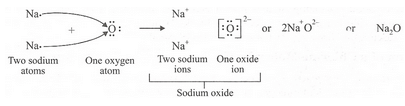(d)I onic compounds are usually hard because their oppositely charged ions attract one another strongly and form a regular crystal structure.

(e) Although solid ionic compounds are made up of ions but they do not conduct electricity in solid state. This is because in the solid ionic compound the ions are held together in fixed positions by strong electrostatic forces and cannot move freely. However, when we dissolve the ionic solid in water or melt it, the crystal structure is broken down and ions become free to move and conduct electricity. Thus, an aqueous solution of an ionic compound conducts electricity because there are plenty of free ions in the solution which are able to conduct electric current.

Question 45:
(a) Write down the electron arrangement in (i) a magnesium atom, and (ii) a chlorine atom.
(b) How many electrons are there in the valence shell of (i) a magnesium atom, and (ii) a chlorine atom ?
(c) Show the formation of magnesium chloride from magnesium and chlorine by the transfer of electrons.
(d) State whether magnesium chloride will conduct electricity or not. Give reason for your answer.
(e) Why are covalent compounds generally poor conductors of electricity ?
Solution :

(a)(i) Magnesium: 2, 8, 2 (ii) Chlorine: 2, 8, 7
(b)(i) 2 (ii) 7
(c)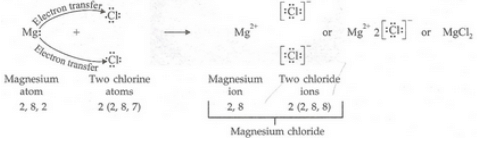(d)Magnesium chloride will conduct electricity because it is an ionic compound .(e) Covalent compounds are generally poor conductors of electricity b ecause they do not contain ions.

(Page No - 171)

Question 67:
Two non-metals combine with each other by the sharing of electrons to form a compound X.
(a) What type of chemical bond is present in X ?
(b) State whether X will have a high melting point or low melting point.
(c) Will it be a good conductor of electricity or not ?
(d) Will it dissolve in an organic solvent or not ?
Solution :

(a) Covalent bond
(b) Low melting point
(c) No
(d) Yes

Question 68:
A metal combines with a non-metal by the transfer of electrons to form a compound Y.
(i) State the type of bonds in Y.
(ii) What can you say about its melting point and boiling point ?
(iii) Will it be a good conductor of electricity ?
(iv) Will it dissolve in an organic solvent or not ?
Solution :

(i) Ionic bond
(ii) High melting point and boiling point
(iii) Yes
(iv) No

Question 69:
The electronic configurations of three elements X, Y and Z are as follows :
X     2, 4
Y     2,1
Z     2, 1
(a) Which two elements will combine to form an ionic compound ?
(b) Which two elements will react to form a covalent compound ?
Solution :

(a) Y and Z will form an ionic compound because an ionic bond is formed when one of the atoms can donate electrons to achieve the inert gas configuration, and the other atom needs electrons to achieve the inert gas configuration. Since Y has 7 and Z has 1 electron in their outermost shell, they would form an ionic bond.
(b) X and Y will react to form a covalent compound because a covalent bond is formed when both the reacting atoms need electrons to achieve the inert gas electron arrangement.

Question 70:
An element A has 4 valence electrons in its atom whereas element B has only one valence electron in its atom. The compound formed by A and B does not conduct electricity. What is the nature of chemical bond in the compound formed ? Give its electron-dot structure.
Solution :

Covalent bond will be formed since covalent compounds are non conductors of electricity.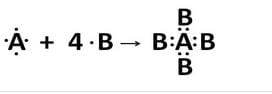Question 71:
In the formation of a compound XY2 atom X gives one electron to each Y atom. What is the nature of bond in XY2 ? Give two properties of XY2.
Solution :

XY2 has ionic bonds.
(i) XY2 would conduct electricity when dissolved in water.
(ii) XY2 would have high melting and boiling point.

Question 72:
An element ‘A’ has two electrons in the outermost shell of its atom and combines with an element ‘B’ having seven electrons in the outermost shell, forming the compound AB2. The compound when dissolved in water conducts electric current. Giving reasons, state the nature of chemical bond in the compound.
Solution :

AB2 forms ionic bonds because an aqueous solution of an ionic compound conducts electricity because there are plenty of free ions in the solution which are able to conduct electric current.

Question 73:
The electronic configurations of two elements A and B are given below :
A    2, 6
B    2, 8, 1
(a) What type of chemical bond is formed between the two atoms of A ?
(b) What type of chemical bond will be formed between the atoms of A and B ?
Solution :

(a) Covalent bond.
(b) Ionic bond .

Question 74:
Four elements A, B, C and D have the following electron arrangements in their atoms :
A    2, 8, 6
B    2, 8, 8
C    2, 8, 8, 1
D    2,1
(a) What type of bond is formed when element C combines with element D ?
(b) Which element is an inert gas ?
(c) What will be the formula of the compound between A and C ?
Solution :

(a) Ionic bond will be formed; element C donates its electron to element D.
(b) B is an inert gas; has complete octet configuration.
(c) C2 A; element A needs two electrons to complete its octet.

Question 75:
An element X of atomic number 12 combines with an element Y of atomic number 17 to form a compound XY2. State the nature of chemical bond in XY2 and show how the electron configurations of X and Y change in the formation of this compound.
Solution :

XYforms ionic bo nd. The electronic configuration of X changes from 2, 8, 2 to 2, 8, it donates its 2 electrons to two Y atoms. Hence, the elec tronic configuration of Y changes f rom 2, 8, 7 to 2, 8, 8 giving the compound XY2.

Question 76:
The electronic configurations of three elements A, B and C are as follows :
A    2, 8, 1
B    2, 8, 7
C    2, 4
(a) Which of these elements is a metal ?
(b) Which of these elements are non-metals ?
(c) Which two elements will combine to form an ionic bond ?
(d) Which two elements will combine to form a covalent bond ?
(e) Which element will form an anion of valency 1 ?
Solution :

(a) A is a metal
(b) B and C are non-metals
(c) A and B combines to form an ionic bond.
(d) B and C combines to form a covalent bond.
(e) B will form an anion with valency 1 since it needs only 1 electron to complete its octet.

Question 77:
The electronic configurations of four particles A, B, C and D are given below :
A    2, 8, 8
B    2, 8, 2
C    2, 6
D    2, 8
Which electronic configuration represents :
(i) magnesium atom ? (ii) oxygen atom ?
(iii) sodium ion ? (iv) chloride ion ?
Solution :

(i) B: Magnesium atom (At. no. = 12)
(ii) C: Oxygen atom (At. no. = 8)
(iii) D: Sodium ion (E.C = 2, 8)
(iv) A: Chloride ion (E.C = 2, 8, 8)

(Page No - 172)

Question 78:
The atomic number of an element X is 12.
(a) What must an atom of X do to attain the nearest inert gas electron configuration ?
(b) Which inert gas is nearest to X ?
Solution :

(a) An atom of X loses 2 electrons to attain the nearest gas electron configuration (2,8).
(b) Neon

Question 79:
The atomic number of an element Y is 16.
(a) What must an atom of Y do to achieve the nearest inert gas electron arrangement ?
(b) Which inert gas is nearest to Y ?
Solution :

(a) A n atom of Y a ccepts 2 electrons to achieve the nearest inert gas electron arrangement (2, 8, 8).
(b) Argon

Question 80:
You can buy solid air-freshners in shops. Do you think these substances are ionic or covalent ? Why ?
Solution :

Solid air-fresheners are covalent compounds because they are volatile compounds with low melting and boiling points.

Question 81:
Give the formulae of the chlorides of the elements X and Y having atomic numbers of 3 and 6 respectively. Will the properties of the two chlorides be similar or different ? Explain your answer.
Solution :

Formula of chloride of element X is XCl
Formula of chloride of element Y is YCl4
The properties of two chlorides will be different because XCl is an i onic chloride whereas YCl4 is a covalent chloride.

The document Lakhmir Singh & Manjit Kaur: Metals and Non-metals, Solutions- 3 | Science Class 10 is a part of the Class 10 Course Science Class 10.
All you need of Class 10 at this link: Class 10

## Science Class 10

78 videos|509 docs|153 tests

## FAQs on Lakhmir Singh & Manjit Kaur: Metals and Non-metals, Solutions- 3 - Science Class 10

 1. What are metals and non-metals?Ans. Metals and non-metals are two categories of elements found on the periodic table. Metals are usually solid, shiny, and good conductors of heat and electricity. They have a high melting and boiling point. Non-metals, on the other hand, may exist in various forms such as solids, liquids, or gases. They are generally dull, poor conductors of heat and electricity, and have a low melting and boiling point.
 2. What are the physical properties of metals?Ans. Metals have several physical properties. They are generally malleable, which means they can be hammered into thin sheets, and ductile, which means they can be drawn into wires. Metals are also good conductors of heat and electricity, have a high density, and are usually solid at room temperature (except for mercury).
 3. What are the physical properties of non-metals?Ans. Non-metals have different physical properties compared to metals. They are generally brittle and cannot be hammered into thin sheets or drawn into wires. Non-metals are poor conductors of heat and electricity, have a low density, and exist in various states at room temperature (solid, liquid, or gas).
 4. Give examples of metals and non-metals.Ans. Examples of metals include iron, copper, aluminum, gold, and silver. Examples of non-metals include carbon, sulfur, oxygen, nitrogen, and hydrogen.
 5. How are metals and non-metals different in terms of chemical properties?Ans. Metals tend to lose electrons to form positively charged ions (cations) during chemical reactions. They have a tendency to oxidize and are good reducing agents. Non-metals, on the other hand, tend to gain electrons to form negatively charged ions (anions) during chemical reactions. They have a tendency to reduce and are good oxidizing agents. This difference in reactivity is due to the difference in electronic configuration between metals and non-metals.

## Science Class 10

78 videos|509 docs|153 testsExplore Courses for Class 10 examSignup to see your scores go up within 7 days! Learn & Practice with 1000+ FREE Notes, Videos & Tests.
10M+ students study on EduRev
Track your progress, build streaks, highlight & save important lessons and more!
Related Searches

,

,

,

,

,

,

,

,

,

,

,

,

,

,

,

,

,

,

,

,

,

,

,

,

;